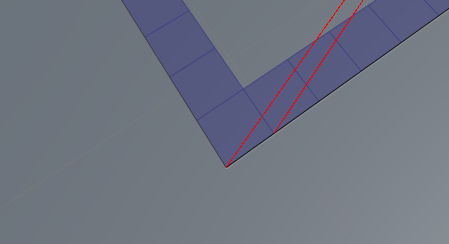# GetContours not closing correctly

While using the GetContours function I noticed that there was 2 points too much in the list.
I was able to visualize this as next:
Lines are being drawn from the center to each Contour point

close up:Code used:
``````public List<Vector3> segments = new List<Vector3>();

public override void OnPostScan()
{
if (AstarPath.active.graphs.Length == 0)
return;

// Get the first graph
NavGraph navmesh = AstarPath.active.graphs;
if (navmesh == null)
return;

segments = GraphUtilities.GetContours(navmesh);
}

private void OnDrawGizmos()
{
if (segments == null || segments.Count == 0)
return;

for (int i = 0; i < 8; i++)
{
Debug.DrawLine(segments[i], Vector3.zero, Color.red, 1);
}
}
``````

After doing some closer investigation it looks like the First point is added 4 times int total ( only 2 times expected )

order of corners being added visualized from bottom to top:

Split corner from previous pictures is top right in this picture

Modified Code:
``````public List<Vector3> segments = new List<Vector3>();

public override void OnPostScan()
{
if (AstarPath.active.graphs.Length == 0)
return;

// Get the first graph
NavGraph navmesh = AstarPath.active.graphs;
if (navmesh == null)
return;

segments = GraphUtilities.GetContours(navmesh);
}

private void OnDrawGizmos()
{
if (segments == null || segments.Count == 0)
return;

for (int i = 0; i < 10; i++)
{
Debug.DrawLine(segments[i], Vector3.zero + new Vector3(0,i,0), Color.red, 1);
}
}
``````

Hi

Thank you for reporting this.
This has already been fixed in the 4.3 beta.

Here is an excerpt from the changelog:

Pathfinding.GraphUtilities.GetContours for grid graphs now simplifies the contour more consistently. Previously there could be one or two additional points where the algorithm started to traverse the contour.

1 Like

Thanks I’ll try it tomorrow